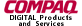gluPwlCurve.3glOpenGL man pages

```

```

## Name

```  gluPwlCurve -	describe a piecewise linear NURBS trimming curve

```

## C Specification

```  void gluPwlCurve( GLUnurbsObj	*nobj,
GLint count,
GLfloat *array,
GLint stride,
GLenum type	)

```

## Parameters

```
nobj	  Specifies the	NURBS object (created with gluNewNurbsRenderer).

count	  Specifies the	number of points on the	curve.

array	  Specifies an array containing	the curve points.

stride  Specifies the	offset (a number of single-precision floating-point
values) between points on the	curve.

type	  Specifies the	type of	curve.	Must be	either GLU_MAP1_TRIM_2 or
GLU_MAP1_TRIM_3.

```

## Description

```  gluPwlCurve describes	a piecewise linear trimming curve for a	NURBS
surface.  A piecewise	linear curve consists of a list	of coordinates of
points in the	parameter space	for the	NURBS surface to be trimmed.  These
points are connected with line segments to form a curve.  If the curve is
an approximation to a	real curve, the	points should be close enough that
the resulting	path appears curved at the resolution used in the
application.

If type is GLU_MAP1_TRIM_2, then it describes	a curve	in two-dimensional (u
and v) parameter space.  If it is GLU_MAP1_TRIM_3, then it describes a
curve	in two-dimensional homogeneous (u, v, and w) parameter space.  Please
trimming curves.

```

## Notes

```  To describe a	trim curve which closely follows the contours of a Nurbs
surface use gluNurbsCurve.

```

```  gluBeginCurve, gluBeginTrim, gluNewNurbsRenderer, gluNurbsCurve